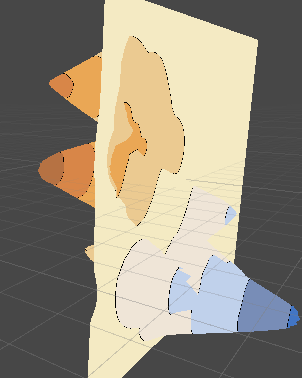# Mesh shader to outline colour changes

Howdy. I’ve written a shader to change colour on a deformable mesh’s Z position. This is to create the effect of topographic/contour maps where the colour changes based on the height of terrain of the mesh.

This is it viewed from the side:And viewed head-on (as it’s meant to be:

What I need is for a black outline between two colour levels. I have done this, but the problem is that the black outline is not pixel perfect (look at the black grouping near the bottom left of the image).

My current shader simply uses if statements to determine which colour level each part should be in, and if it’s within a certain threshold BETWEEN two colour layers, make it black. Obviously this is wrong.

``````Shader "Unlit/MapEditorShader"
{
Properties {

_Level8Color ("Level8Color", Color) = (0.8,0.9,0.9,1)
_Level8 ("Level8", Float) = 30
_Level7Color ("Level7Color", Color) = (0.8,0.9,0.9,1)
_Level7 ("Level7", Float) = 30
_Level6Color ("Level6Color", Color) = (0.8,0.9,0.9,1)
_Level6 ("Level6", Float) = 30
_Level5Color ("Level5Color", Color) = (0.8,0.9,0.9,1)
_Level5 ("Level5", Float) = 30
_Level4Color ("Level4Color", Color) = (0.8,0.9,0.9,1)
_Level4 ("Level4", Float) = 30
_Level3Color ("Level3Color", Color) = (0.75,0.53,0,1)
_Level3 ("Level3", Float) = 20
_Level2Color ("Level2Color", Color) = (0.69,0.63,0.31,1)
_Level2 ("Level2", Float) = 10
_Level1Color ("Level1Color", Color) = (0.65,0.86,0.63,1)
_Level1 ("Level1", Float) = 0
_Level0Color ("Level0Color", Color) = (0.37,0.78,0.92,1)
_OutlineColor ("OutlineColor", Color) = (0,0,0,1)
_Slope ("Slope Fader", Range (0,1)) = 0

}
Tags { "RenderType" = "Diffuse" }
CGPROGRAM
#pragma surface surf SimpleLight
struct Input {
float3 customColor;
float3 worldPos;
};

half4 LightingSimpleLight (SurfaceOutput s, half3 lightDir, half atten) {
half4 c;
c.rgb = s.Albedo * 0.8;
c.a = s.Alpha;
return c;
}

float _Level8;
float4 _Level8Color;
float _Level7;
float4 _Level7Color;
float _Level6;
float4 _Level6Color;
float _Level5;
float4 _Level5Color;
float _Level4;
float4 _Level4Color;
float _Level3;
float4 _Level3Color;
float _Level2;
float4 _Level2Color;
float _Level1;
float4 _Level1Color;
float _Slope;
float4 _Level0Color;
float4 _OutlineColor;

void surf (Input IN, inout SurfaceOutput o) {
//at breakpoints we want a black line
if((IN.worldPos.z > _Level8 - _Slope && IN.worldPos.z < _Level8 + _Slope)||
(IN.worldPos.z > _Level7 - _Slope && IN.worldPos.z < _Level7 + _Slope)||
(IN.worldPos.z > _Level6 - _Slope && IN.worldPos.z < _Level6 + _Slope)||
(IN.worldPos.z > _Level5 - _Slope && IN.worldPos.z < _Level5 + _Slope)||
(IN.worldPos.z > _Level4 - _Slope && IN.worldPos.z < _Level4 + _Slope)||
(IN.worldPos.z > _Level3 - _Slope && IN.worldPos.z < _Level3 + _Slope)||
(IN.worldPos.z > _Level2 - _Slope && IN.worldPos.z < _Level2 + _Slope)||
(IN.worldPos.z > _Level1 - _Slope && IN.worldPos.z < _Level1 + _Slope))
o.Albedo = _OutlineColor;
else if (IN.worldPos.z > _Level8)
o.Albedo = _Level8Color;
else if (IN.worldPos.z > _Level7)
o.Albedo = _Level7Color;
else if (IN.worldPos.z > _Level6)
o.Albedo = _Level6Color;
else if (IN.worldPos.z > _Level5)
o.Albedo = _Level5Color;
else if (IN.worldPos.z > _Level4)
o.Albedo = _Level4Color;
else if (IN.worldPos.z > _Level3)
o.Albedo = _Level3Color;
else if (IN.worldPos.z > _Level2)
o.Albedo = _Level2Color;
else if (IN.worldPos.z > _Level1)
o.Albedo = _Level1Color;
else
o.Albedo = _Level0Color;

}
ENDCG
}
Fallback "Opaque"
}
``````

Any suggestions would be greatly appreciated.

Because your depth values don’t come from a texture, you can scale the slope by its screen-space derivative to get uniform width across the line;

``````float slope = fwidth (IN.worldPos.z) * _Slope;

if((IN.worldPos.z > _Level8 - slope && IN.worldPos.z < _Level8 + slope)||
(IN.worldPos.z > _Level7 - slope && IN.worldPos.z < _Level7 + slope)||
(IN.worldPos.z > _Level6 - slope && IN.worldPos.z < _Level6 + slope)||
(IN.worldPos.z > _Level5 - slope && IN.worldPos.z < _Level5 + slope)||
(IN.worldPos.z > _Level4 - slope && IN.worldPos.z < _Level4 + slope)||
(IN.worldPos.z > _Level3 - slope && IN.worldPos.z < _Level3 + slope)||
(IN.worldPos.z > _Level2 - slope && IN.worldPos.z < _Level2 + slope)||
(IN.worldPos.z > _Level1 - slope && IN.worldPos.z < _Level1 + slope))
o.Albedo = _OutlineColor;
``````

There are definitely cleaner ways to write what you are attempting, but if it works it works.